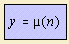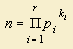# Möbius function

## discrete curvewhere the Möbius function μ is defined as follows:let a natural number n to be written as a multiplication of primes:Then μ(n) = (-1)r where k1= .....=kr=1; μ(1) = 1; μ(n) = 0 otherwise.

It follows that μ = -1 for each prime. This extraordinary function is used in the Prime Number Theorem.